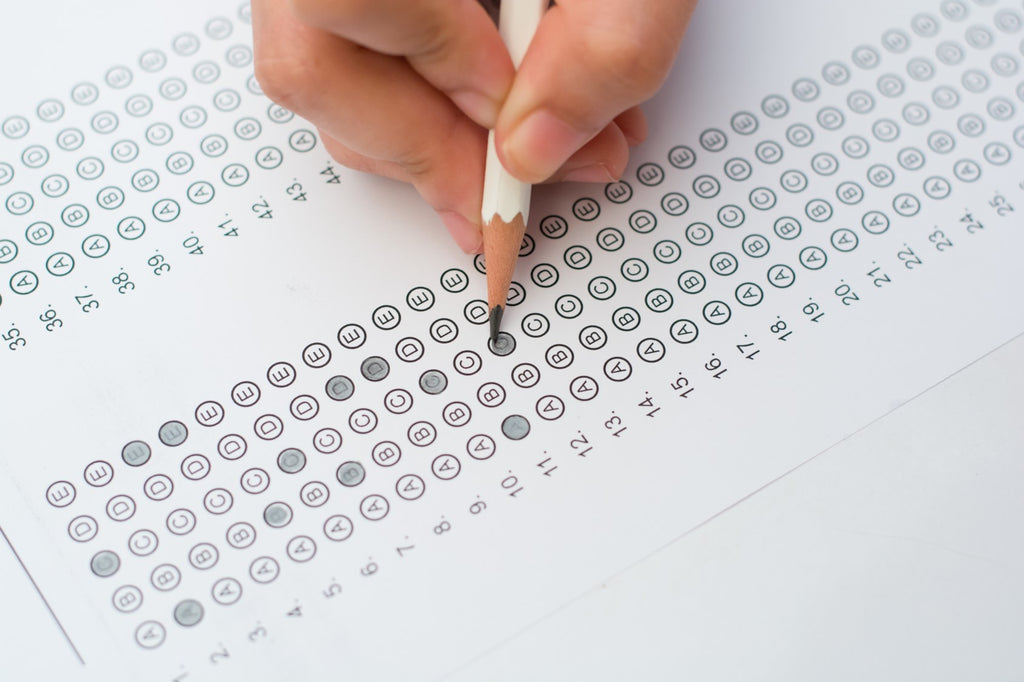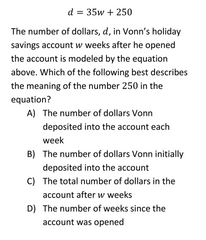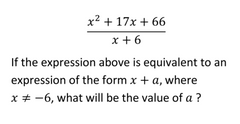# Common SAT Math problems and how to solve them: Part (I)# Common SAT Math problems and how to solve them

Thinking of taking SATs but not sure what to expect in the SAT Math?

Currently preparing for SAT Math and want to know which skills are a must to ace the test?

Finished preparing for SATs and want to quickly glance through some common Math problems?

Whichever stage of SAT Math preparation you are in right now, the following compilation of SAT Math problems and solutions will give you something to take away.

If you don’t know it already, SAT Math tests students in different areas of mathematics, ranging from algebra and arithmetic to statistics, geometry and trigonometry.

These areas are considered pivotal to succeeding in college and beyond.

Each of these questions tests students’ mastery of core concepts, critical thinking skills, and ability to apply the concepts in creative problem solving.

While the topics and the types of questions are numerous, there are a few types of problems that have appeared frequently over the years Part (I).

This article takes a closer look at such questions and discusses the best approach to solve them.

### 1. Algebra – Interpretation of Slope or Y-intercept from a Linear ModelAs you can see, the given equation is written in the form y = mx + c, where m represents the slope of the line and c denotes the y-intercept (the point where the line intersects the Y-axis).

You can picture the number of dollars, d, plotted in the Y-axis and number of weeks, w, plotted in the X-axis.

Then, the aforementioned equation will represent a line with slope 35 intersecting the d-axis at (0, 250), as shown in the figure below.Figure sketched using Desmos

You can see that 200 is the amount of dollars in Vonn’s holiday savings account at week 0 (w = 0). This is the initial amount Vonn had deposited in his savings account. So, the answer is option B.

A quick question—what if the question had asked you to interpret the number 35?

Well, since the number 35 represents the slope, it is the change in the value of d for every unit increase in the value of w.

This means the value of dollar deposited (d) in the savings account is 35 for every unit increase in the number of weeks.

Thus, 35 represents the number of dollars Vonn deposited into the account each week (option A).

### 2. Rate, Ratio and Proportion – Unit ConversionThe rate in the question is given in dollars per cubic yard. The question asks you to find the price for 54 cubic feet. So, the first step to solving this problem is converting 54 cubic feet to cubic yard.

If you are worried that you may not always remember the rates of unit conversion, fret not! Collegeboard does not expect you to memorize the conversion rates for different units in SAT Math.

Instead, it provides you that information in the question, and tests your ability to make its proper use for solving the problem.

In this question, it is given that 1 yard equals to 3 feet. This means 1 foot (singular of feet) equals to 1/3 yard.

Using this equivalence, we can convert 54 cubic feet to cubic yard by multiplying 54 by 1/3 three times.

Three times? Why?

Because the unit “cubic feet” contains “feet” multiplied three times and each feet has to multiplied by 1/3 when converting it to yard.

In this way, 54 cubic feet equals to 54*1/3*1/3*1/3 cubic yard, which is 2 cubic yards. Since the price of one cubic yard is \$30, the price of 2 cubic yards therefore equals to 2*30 = \$60, which is option B.

### 3. Algebra – Equivalent ExpressionsConverting an algebraic expression to its equivalent form is one of the most tested skills in algebra. In this case, the numerator has an algebraic expression in the expanded form, and you are supposed to factorize it.

In case you are not already familiar, when we have algebraic expression in expanded form with 3 terms, the first strategy would be to check if there are two perfect squares floating around.

That way, we can check if the terms are in the form of a2 ± 2ab + b2, which can be succinctly written as (a ± b)2. In this case, the first term x2 is a perfect square, but the other two aren’t. So, this expression is clearly not in the form we suspect.

Our next strategy would be to break the middle term such that we have four terms instead of three and then we can factor out common term in each two terms.

So how do we apply this method?

Well, we can use the following steps:

1. Prime factorize the constant term, 66. That would be 6*11*1.
2. Select two numbers whose product gives you the constant term and whose sum gives you the middle term. (Note that we want sum of those two numbers because the constant term is positive in this case. If the constant term was negative, we would have looked for two numbers whose difference would give the middle term.) That would be 6 and 11 as 6 times 11 gives you the constant term 66, and the sum 6 + 11 equals to 17, the coefficient of the middle term.
3. Break down the algebraic expression with three terms to one with four terms. Thus, x2 + 17x + 66 = x2 + 6x + 11x + 66
4. Find the common factor in each two terms, i.e. first and second term, third and fourth term. This gives x2 + 6x + 11x + 66 = x (x +6) + 11 (x + 6) = (x + 6) (x + 11).

Now we can see that (x + 6) is a common factor in both numerator and denominator, so we can cancel that out. Hence, we are left with (x + 11) only. Comparing (x + 11) with x + a, we can read off a= 11, which is our answer.

In the next part, we will be introducing 4 more types of common SAT math problems:

1. Functions and Graphs – Formulating and Solving Equations
2. T
rigonometry – Using SOH CAH TOA in Right Triangle
3.
Geometry – Calculating Side Lengths using Similar Triangles
4.
Statistics – Central Tendency and Data Dispersion

Stay tuned till Part (II)